Concepts

Class 10
Chapter 3 Class 10 - Metals and Non-Metals

# Different States of Metals and Non Metals :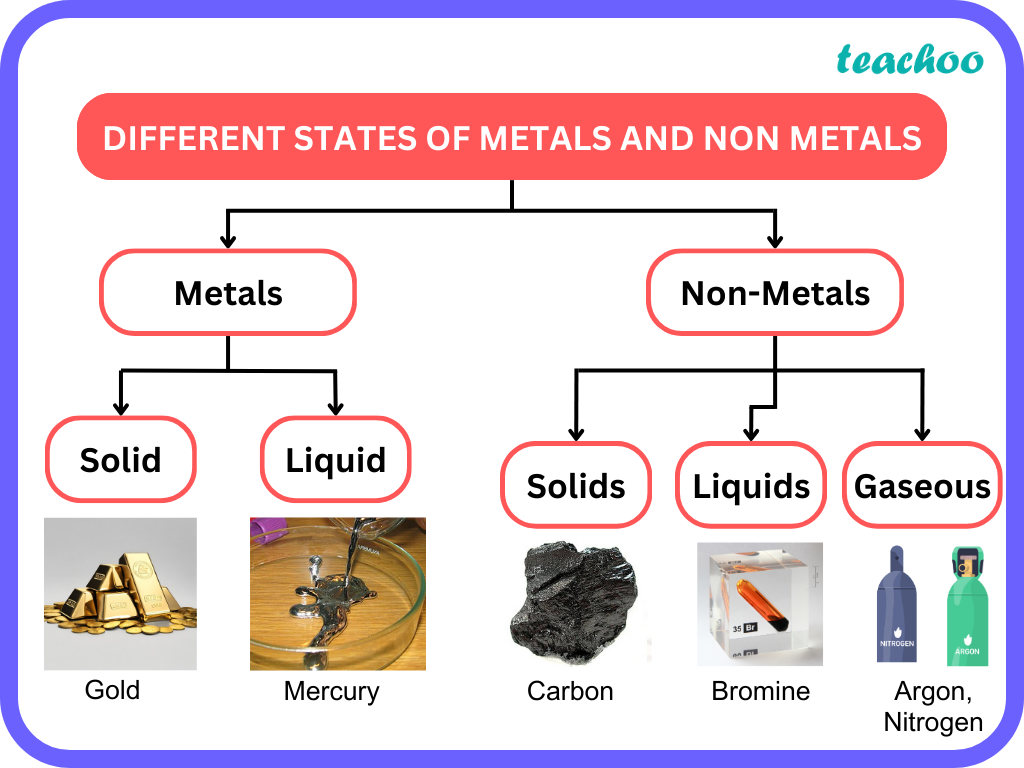# Uses of metals

Metals have various uses in all fields depending upon their physical and chemical properties:

• Conductors of electricity like Copper and Aluminium are used to make wires
• Though silver is also an extremely good conductor of electricity, wires are not made from silver since it is very expensive .
• Iron , Copper , and Aluminium are used to make utensils and equipment due to their malleability, hardness, and good thermal conductivity.
• Zinc , Copper and Aluminium are used as protective metals to prevent other metals from corrosion.
• Zinc is used to protect Iron from rusting . The process of placing a protective coating of Zinc on Iron is known as Galvanisation .
• Copper and Aluminium form a protective oxide layer on top and prevent the rest of the metal from corrosion.
• Aluminium is also used to make foils , packaging in medicine, cigarettes and food material.
• Gold, Silver, Platinum are used to make jewellery
• Mercury is used in thermometers.
• Nickel, Titanium, Zirconium, Uranium are used in Nuclear Energy .

Energy Levels or Shells

We know that an atom is made up of protons,neutrons and electrons .

• Protons and neutrons are present in the nucleus of an atom. However, electrons move around the nucleus in orbits
• These orbits are called energy levels or shells .

### What is the Number of Electrons in a Shell?

• Each Energy Shell has a different number of electrons and corresponds to a different energy level.
• The innermost shell of an atom is called the K shell. Moving outwards, the shells are named, L, M, N and so on.
• As we move outwards from the nucleus, the energy and number of electrons in each shell increases .

 Shell Name Other Name Max No of Electrons K shell n=1 2 L Shell n=2 8 M Shell n=3 18 N Shell n=4 32

### How is the Maximum Number of Electrons in a shell Calculated?

It is calculated by Using formula 2n 2

Example

For K shell.n=1

So number of Electrons = 2n 2 = 2*1 2 = 2*1 = 2

Similarly for L shell.n=2

So number of Electrons = 2*n 2 = 2*2 2 = 2*4 = 8

Similarly for M shell.n=3

So number of Electrons = 2*n 2 = 2*3 2   = 2*9 = 18

Similarly for N shell.n=4

So number of Electrons = 2*n 2 = 2*4 2 = 2*16 = 32

### What are Valency and Valence Electrons?

• The electrons in the outermost shell of an atom are called valence electrons.

If no. of Valence electron <4,

Valency= no. of valence electrons

If no. of Valence electron >4,

Valency= 8- no. of valence electrons

If it is a noble metal, Valency=0

For Example :

1. Helium has 2 Electrons
• All these are in K Shell
• K shell is the outermost shell
• It can hold a maximum of  2 electrons.

Hence, Helium has 2 Valence Electrons. Valency=0.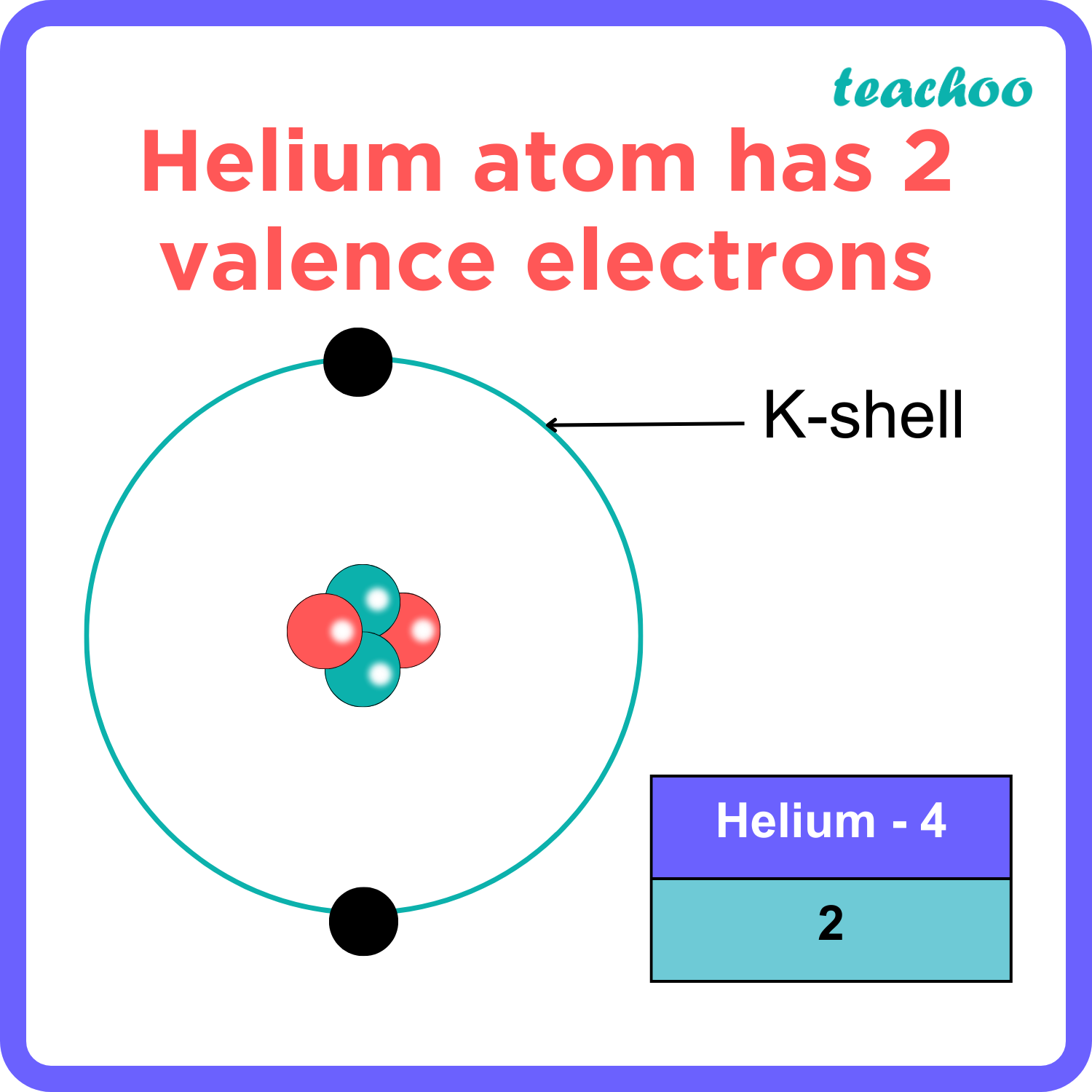1. Sodium has 11 Electrons
• Two of these are held in K Shell
• 8 of these are held in L Shell
• Remaining 1 is held in M Shell.

Hence,  Sodium has 1 Valence Electron.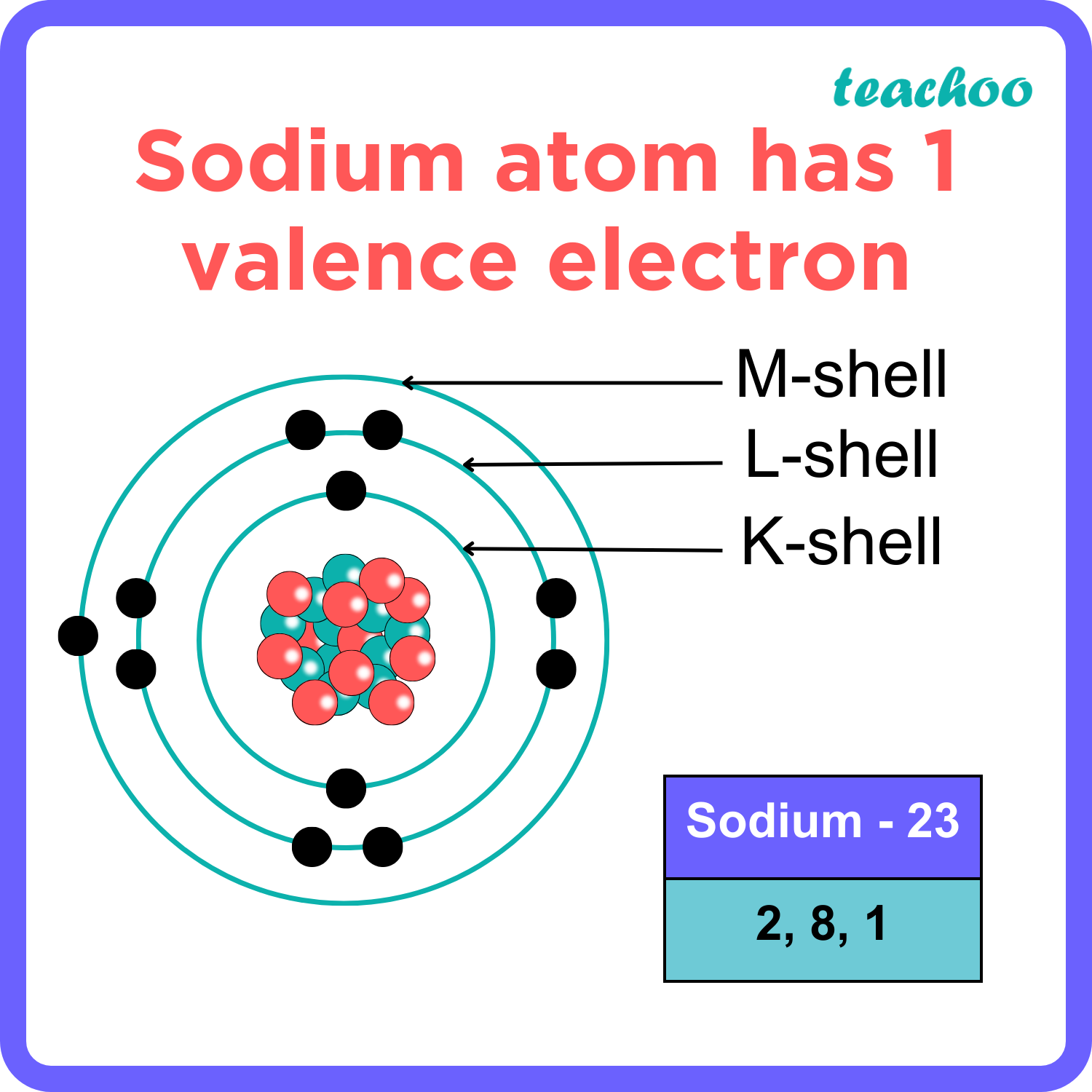1. Chlorine has 17 Electrons.
• Two of these are held in K Shell
• 8 of these are held in L Shell
• Remaining 7 are held in M Shell.

Hence,  Chlorine has 7 Valence Electrons. Valency=1 (8-7)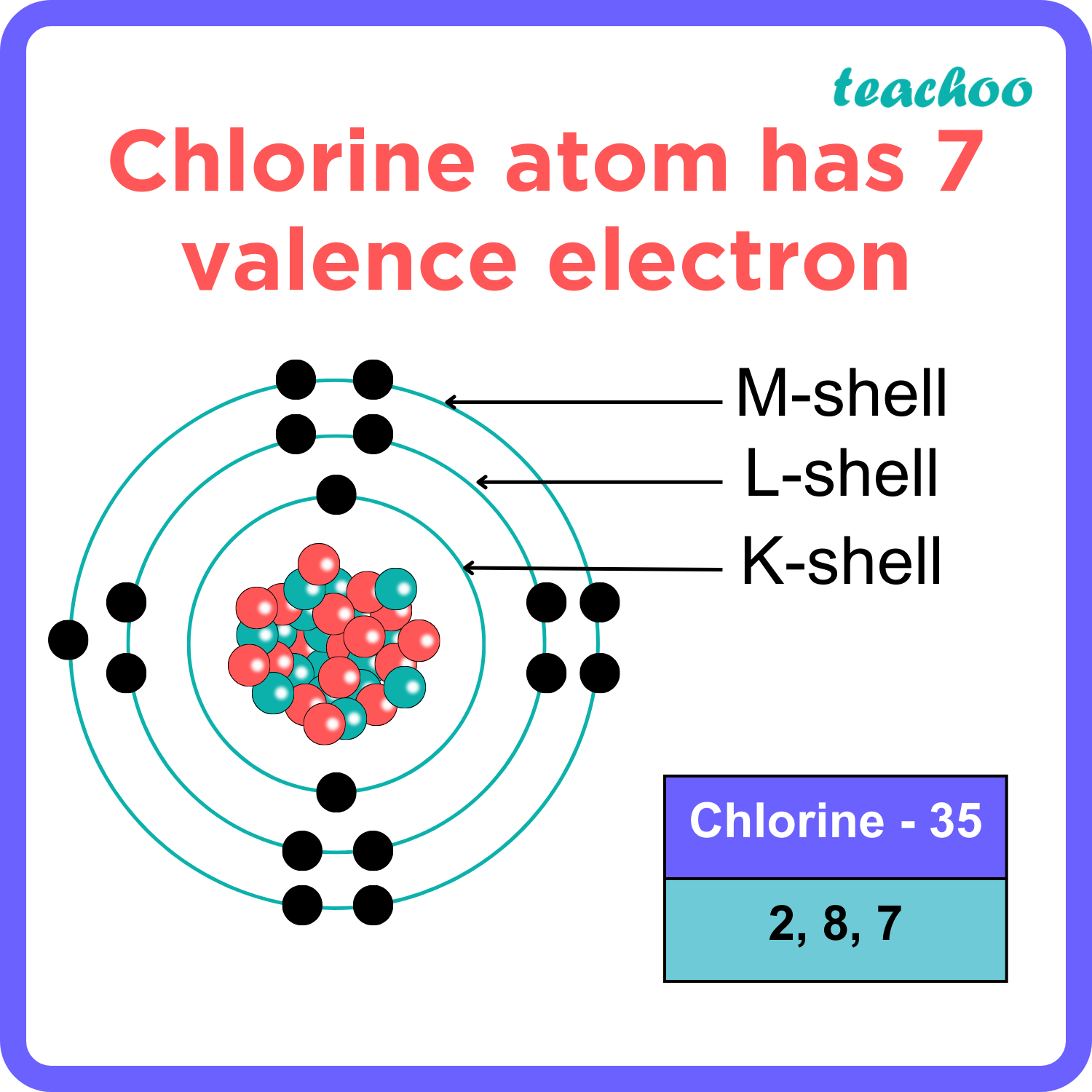Why are Valence Electrons important?

• Valence Electrons are the electrons from the outermost shells
• Only these electrons take part in chemical reactions .
• Elements with a full outermost shell do not react with other elements.
• These elements are known as Noble/Inert gases.

For Example,

• Helium has 2 Elements in its outer shell.
• K Shell can only hold 2 electrons.
• Hence, there are no free electrons which take part in chemical reactions.
• Valency of these elements is 0.

Examples:

 Element Valency Helium 0 Neon 0 Argon 0 Krypton 0 Xenon 0 Radon 0

### What is an Ion?

• A charged atom is called an ion.
• It may be positively or negatively charged.
• A Positively charged ion is called a Cation whereas a negatively charged ion is called an anion .

### How are Ions formed?

We know that an atom contains protons,neutrons and electrons.

• Protons are positively charged, Electrons are Negatively charged and Neutrons are neutrally charged.
• In an atom, the no of protons and electrons are equal such that an atom is neutral .
• When an atom loses electrons , it becomes positively charged
• Similarly, when an atom gains electrons , it becomes negatively charged .

### Formation of an anion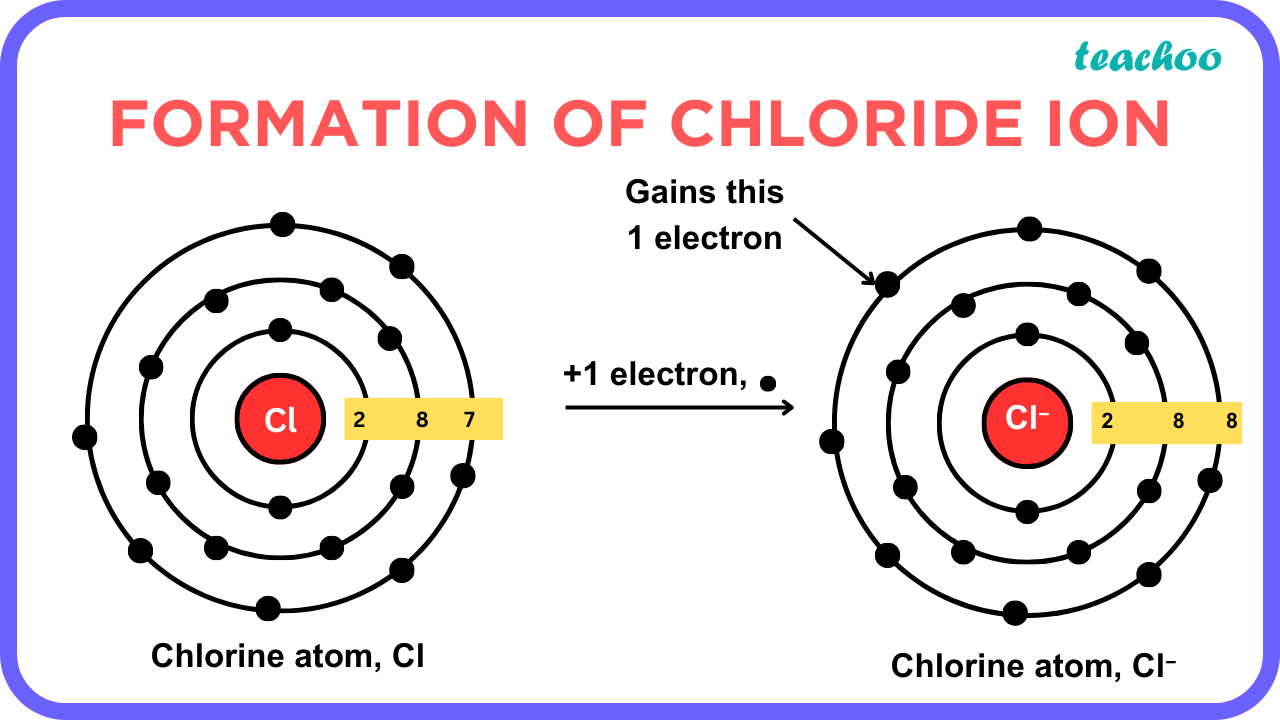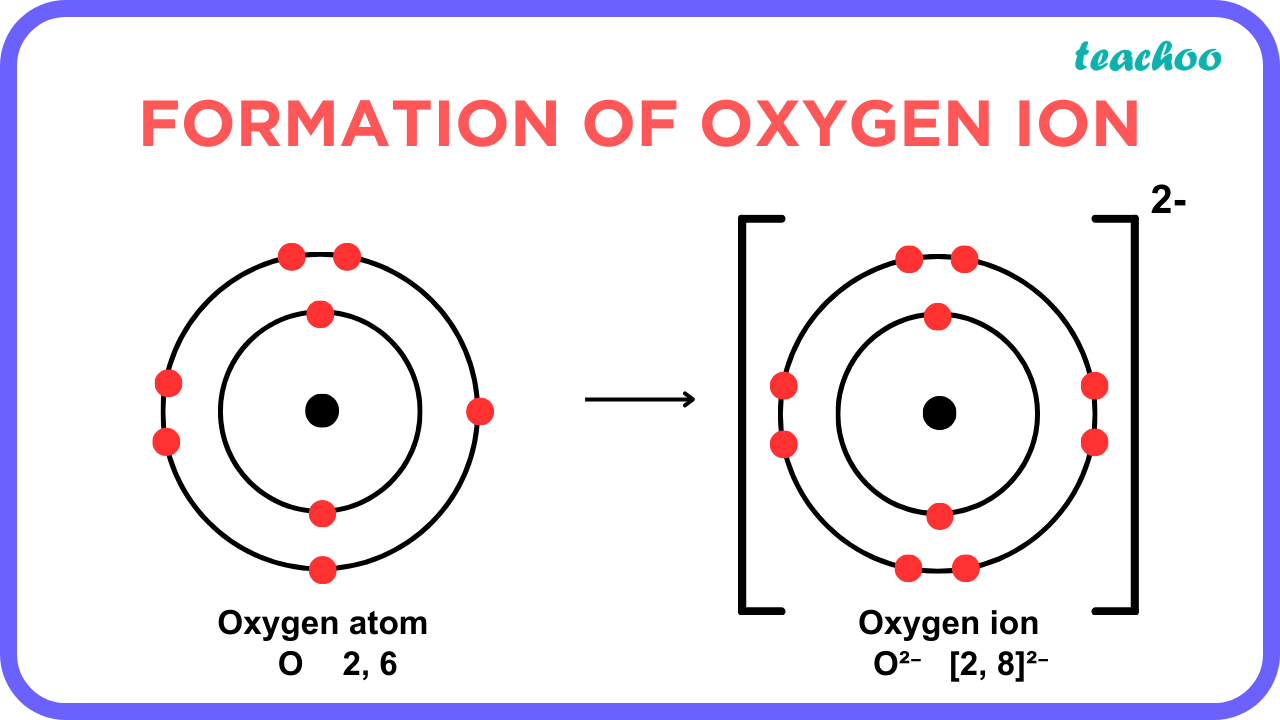Learn in your speed, with individual attention - Teachoo Maths 1-on-1 Class